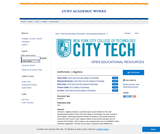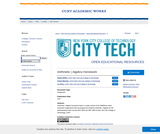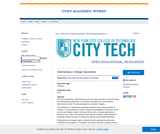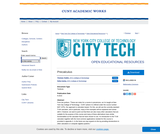# 4 Results

View
Selected filters:
• New York City College of TechnologyOnly Sharing Permitted
CC BY-NC-ND
Rating

Arithmetic | Algebra provides a customized open-source textbook for the math developmental students at New York City College of Technology. The book consists of short chapters, addressing essential concepts necessary to successfully proceed to credit-level math courses. Each chapter provides several solved examples and one unsolved “Exit Problem”. Each chapter is also supplemented by its own WeBWork online homework assignment. The book can be used in conjunction with WeBWork for homework (online) or with the Arithmetic | Algebra Homework handbook (traditional). The content in the book, WeBWork and the homework handbook are also aligned to prepare students for the CUNY Elementary Algebra Final Exam (CEAFE).

Subject:
Algebra
Material Type:
Textbook
Provider:
Provider Set:
New York City College of Technology
Author:
Holly Carley
Lin Zhou
Marianna Bonanome
Samar ElHitti
01/01/2017Only Sharing Permitted
CC BY-NC-ND
Rating

Arithmetic | Algebra Homework book is a static version of the WeBWork online homework assignments that accompany the textbook Arithmetic | Algebra for the developmental math courses MAT 0630 and MAT 0650 at New York City College of Technology, CUNY.

Subject:
Algebra
Material Type:
Homework/Assignment
Provider:
Provider Set:
New York City College of Technology
Author:
Ariane Masouda
Lin Zhou
Samar ElHitti
01/01/2017Conditional Remix & Share Permitted
CC BY-NC-SA
Rating

This text is intended for a brief introductory course in plane geometry. It covers the topics from elementary geometry that are most likely to be required for more advanced mathematics courses. The only prerequisite is a semester of algebra.

The emphasis is on applying basic geometric principles to the numerical solution of problems. For this purpose the number of theorems and definitions is kept small. Proofs are short and intuitive, mostly in the style of those found in a typical trigonometry or precalculus text. There is little attempt to teach theorem-proving or formal methods of reasoning. However the topics are ordered so that they may be taught deductively.

The problems are arranged in pairs so that just the odd-numbered or just the even-numbered can be assigned. For assistance, the student may refer to a large number of completely worked-out examples. Most problems are presented in diagram form so that the difficulty of translating words into pictures is avoided. Many problems require the solution of algebraic equations in a geometric context. These are included to reinforce the student's algebraic and numerical skills, A few of the exercises involve the application of geometry to simple practical problems. These serve primarily to convince the student that what he or she is studying is useful. Historical notes are added where appropriate to give the student a greater appreciation of the subject.

This book is suitable for a course of about 45 semester hours. A shorter course may be devised by skipping proofs, avoiding the more complicated problems and omitting less crucial topics.

Subject:
Geometry
Material Type:
Textbook
Provider:
Provider Set:
New York City College of Technology
Author:
Henry Africk
01/01/2013Conditional Remix & Share Permitted
CC BY-NC-SA
Rating

From the preface, "These are notes for a course in precalculus, as it is taught at New York City College of Technology - CUNY (where it is offered under the course number MAT 1375). Our approach is calculator based. For this, we will use the currently standard TI-84 calculator, and in particular, many of the examples will be explained and solved with it. However, we want to point out that there are also many other calculators that are suitable for the purpose of this course and many of these alternatives have similar functionalities as the calculator that we have chosen to use. An introduction to the TI-84 calculator together with the most common applications needed for this course is provided in appendix A. In the future we may expand on this by providing introductions to other calculators or computer algebra systems."

I Functions and graphs

1 The absolute value
2 Lines and functions
3 Functions by formulas and graphs
4 Introduction to the TI-84
5 Basic functions and transformations
6 Operations on functions
7 The inverse of a function
II Polynomials and rational functions

8 Dividing polynomials
9 Graphing polynomials
10 Roots of polynomials
11 Rational functions
12 Polynomial and rational inequalities
III Exponential and logarithmic functions

13 Exponential and logarithmic functions
14 Properties of exp and log
15 Applications of exp and log
16 Half-life and compound interest
IV Trigonometric functions

17 Trigonometric functions
18 Addition of angles and multiple angles
19 Inverse trigonometric functions
20 Trigonometric equations
V Complex numbers, sequences, and the binomial theorem

21 Complex numbers
22 Vectors in the plane
23 Sequences and series
24 The geometric series
25 The binomial theorem

Subject:
Calculus
Material Type:
Textbook
Provider: